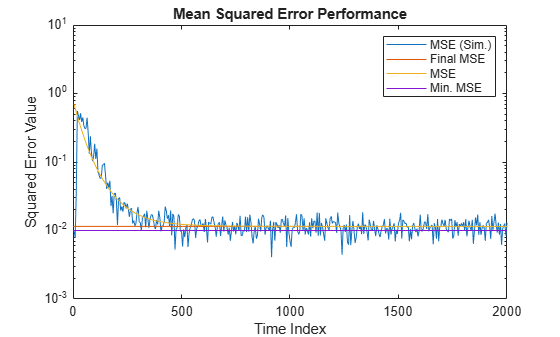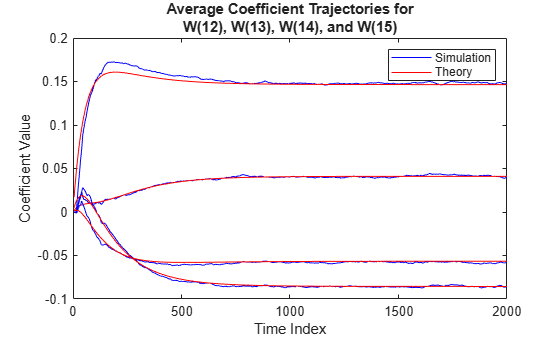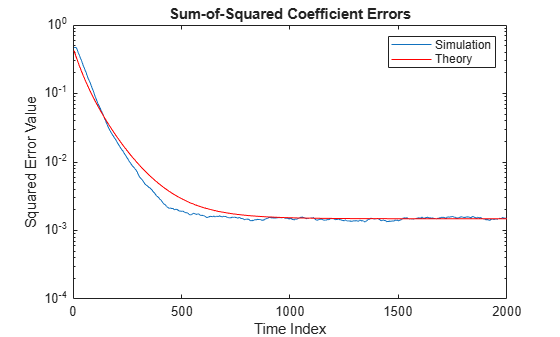Main Content

# msepred

Predicted mean squared error for LMS adaptive filter

## Syntax

``[mmseemse] = msepred(lmsFilt,x,d)``
``[mmseemse,meanw,mse,tracek] = msepred(lmsFilt,x,d)``
``[mmseemse,meanw,mse,tracek] = msepred(lmsFilt,x,d,m)``

## Description

example

````[mmseemse] = msepred(lmsFilt,x,d)` predicts the steady-state values at convergence of the minimum mean squared error, `mmse`, and the excess mean squared error, `emse`, given the input and the desired response signal sequences in `x` and `d` and the quantities in the `dsp.LMSFilter` System object™, `lmsFilt`.```

example

````[mmseemse,meanw,mse,tracek] = msepred(lmsFilt,x,d)` also computes `meanw`, the sequence of means of the coefficient vectors, `mse`, sequence of mean-squared errors, and `tracek`, the sequence of total coefficient error powers. ```

example

````[mmseemse,meanw,mse,tracek] = msepred(lmsFilt,x,d,m)` specifies an optional decimation factor for computing `meanw`, `mse`, and `tracek`. If `m` > 1, every `m`th predicted value of each of these sequences is saved. If omitted, the value of `m` is the default, which is one.```

## Examples

collapse all

The mean squared error (MSE) measures the average of the squares of the errors between the desired signal and the primary signal input to the adaptive filter. Reducing this error converges the primary input to the desired signal. Determine the predicted value of MSE and the simulated value of MSE at each time instant using the `msepred` and `msesim` functions. Compare these MSE values with each other and with respect to the minimum MSE and steady-state MSE values. In addition, compute the sum of the squares of the coefficient errors given by the trace of the coefficient covariance matrix.

Note: If you are using R2016a or an earlier release, replace each call to the object with the equivalent step syntax. For example, `obj(x)` becomes `step(obj,x)`.

Initialization

Create a `dsp.FIRFilter` System object™ that represents the unknown system. Pass the signal, `x`, to the FIR filter. The output of the unknown system is the desired signal, `d`, which is the sum of the output of the unknown system (FIR filter) and an additive noise signal, `n`.

```num = fir1(31,0.5); fir = dsp.FIRFilter('Numerator',num); iir = dsp.IIRFilter('Numerator',sqrt(0.75),... 'Denominator',[1 -0.5]); x = iir(sign(randn(2000,25))); n = 0.1*randn(size(x)); d = fir(x) + n; ```

LMS Filter

Create a `dsp.LMSFilter` System object to create a filter that adapts to output the desired signal. Set the length of the adaptive filter to 32 taps, step size to 0.008, and the decimation factor for analysis and simulation to 5. The variable `simmse` represents the simulated MSE between the output of the unknown system, `d`, and the output of the adaptive filter. The variable `mse` gives the corresponding predicted value.

```l = 32; mu = 0.008; m = 5; lms = dsp.LMSFilter('Length',l,'StepSize',mu); [mmse,emse,meanW,mse,traceK] = msepred(lms,x,d,m); [simmse,meanWsim,Wsim,traceKsim] = msesim(lms,x,d,m);```

Plot the MSE Results

Compare the values of simulated MSE, predicted MSE, minimum MSE, and the final MSE. The final MSE value is given by the sum of minimum MSE and excess MSE.

```nn = m:m:size(x,1); semilogy(nn,simmse,[0 size(x,1)],[(emse+mmse)... (emse+mmse)],nn,mse,[0 size(x,1)],[mmse mmse]) title('Mean Squared Error Performance') axis([0 size(x,1) 0.001 10]) legend('MSE (Sim.)','Final MSE','MSE','Min. MSE') xlabel('Time Index') ylabel('Squared Error Value')```The predicted MSE follows the same trajectory as the simulated MSE. Both these trajectories converge with the steady-state (final) MSE.

Plot the Coefficient Trajectories

`meanWsim` is the mean value of the simulated coefficients given by `msesim`. `meanW` is the mean value of the predicted coefficients given by `msepred`.

Compare the simulated and predicted mean values of LMS filter coefficients 12,13,14, and 15.

```plot(nn,meanWsim(:,12),'b',nn,meanW(:,12),'r',nn,... meanWsim(:,13:15),'b',nn,meanW(:,13:15),'r') PlotTitle ={'Average Coefficient Trajectories for';... 'W(12), W(13), W(14), and W(15)'}```
```PlotTitle = 2x1 cell {'Average Coefficient Trajectories for'} {'W(12), W(13), W(14), and W(15)' } ```
```title(PlotTitle) legend('Simulation','Theory') xlabel('Time Index') ylabel('Coefficient Value')```In steady state, both the trajectories converge.

Sum of Squared Coefficient Errors

Compare the sum of the squared coefficient errors given by `msepred` and `msesim`. These values are given by the trace of the coefficient covariance matrix.

```semilogy(nn,traceKsim,nn,traceK,'r') title('Sum-of-Squared Coefficient Errors') axis([0 size(x,1) 0.0001 1]) legend('Simulation','Theory') xlabel('Time Index') ylabel('Squared Error Value')```## Input Arguments

collapse all

LMS adaptive filter, specified as a `dsp.LMSFilter` System object.

Input signal, specified as a scalar, column vector, or matrix. Columns of the matrix `x` contain individual input signal sequences. The input, `x`, and the desired signal, `d`, must have the same size, data type, and complexity.

Data Types: `single` | `double`

Desired response signal, specified as a scalar, column vector, or matrix. Columns of the matrix `d` contain individual desired signal sequences. The input, `x`, and the desired signal, `d`, must have the same size, data type, and complexity.

Data Types: `single` | `double`

Decimation factor, specified as a positive scalar. Every `m`th predicted value of the 3rd, 4th, and 5th predicted sequences output is saved into the corresponding output arguments, `meanw`, `mse`, and `tracek`. If `m` equals 1, every value of these sequences is saved.

`m` must be a factor of the input frame size.

Data Types: `single` | `double` | `int8` | `int16` | `int32` | `int64` | `uint8` | `uint16` | `uint32` | `uint64` | `logical`

## Output Arguments

collapse all

Minimum mean squared error (mmse), returned as a scalar. This parameter is estimated using a Wiener filter. The Wiener filter minimizes the mean squared error between the desired signal and the input signal filtered by the Wiener filter. A large value of the mean squared error indicates that the adaptive filter cannot accurately track the desired signal. The minimal value of the mean squared error ensures that the adaptive filter is optimal. The minimum mean squared error between a particular frame of the desired signal and the filtered signal is computed as the variance between the two frames of signals. The `msepred` function outputs the average of the mmse values for all the frames. For more details on how this parameter is calculated, see Algorithms.

Data Types: `single` | `double`

Excess mean squared error, returned as a scalar. This error is the difference between the mean squared error introduced by adaptive filters and the minimum mean squared error produced by corresponding Wiener filter. For details on how this parameter is calculated, see Algorithms.

Data Types: `single` | `double`

Sequence of coefficient vector means of the adaptive filter at each time instant, returned as a matrix. The columns of this matrix contain predictions of the mean values of the LMS adaptive filter coefficients at each time instant. If the decimation factor, `m` equals 1, the dimensions of `meanw` is M-by-N. M is the frame size (number of rows) of the input signal, `x`. N is the length of the FIR filter weights vector, specified by the `Length` property of the `lmsFilt` System object. If `m` > 1, the dimensions of `meanw` is M/`m`-by-N.

For details on how this parameter is calculated, see Algorithms.

Data Types: `double`

Predictions of the mean squared error of the LMS adaptive filter at each time instant, returned as a column vector. If the decimation factor, `m` equals 1, the length of `mse` equals the frame size (number of rows) of the input signal, M. If `m` > 1, the length of `mse` equals M/`m`.

For details on how this parameter is calculated, see Algorithms.

Data Types: `double`

Predictions of the total coefficient error power of the LMS adaptive filter at each time instant, returned as a column vector. If the decimation factor, `m` equals 1, the length of `tracek` equals the frame size (number of rows) of the input signal, given by size(`x`,1). If `m` > 1, the length of `tracek` equals the ratio of input frame size and the decimation factor, `m`.

For details on how this parameter is calculated, see Algorithms.

Data Types: `double`

## Algorithms

collapse all

### Minimum Mean Squared Error (mmse)

The `msepred` function computes the minimum mean-squared error (mmse) using the following equation:

`$mmse=\frac{\sum _{i=1}^{N}\mathrm{var}\left({d}_{i}-W\left({x}_{i}\right)\right)}{N}$`

where,

• N –– Number of frames in the input signal, `x`.

• di –– ith frame (column) of the desired signal.

• xi –– ith frame (column) of the input signal.

• W(xi) –– Output of the Wiener filter.

• `var` –– Variance

### Excess Mean Squared Error (emse)

The `msepred` function computes the steady-state excess mean squared error using the following equations:

`$\begin{array}{l}emse=K\lambda ,\\ K=\frac{B}{I\left(L\right)-A},\\ B={\mu }^{2}.mmse.\lambda \text{'},\\ A=I\left(L\right)-2\mu Lam+{\mu }^{2}\left(La{m}^{2}\left(kurt+2\right)+\lambda \lambda \text{'}\right).\end{array}$`

where,

• K –– Final values of transformed coefficient variances.

• λ –– Column vector containing the eigenvalues of the input autocorrelation matrix.

• λ' –– Transpose of λ.

• B –– MSE analysis driving term.

• L –– Length of the FIR adaptive filter, given by `lmsFilt.Length`.

• I(L) –– L-by-L identity matrix.

• A –– MSE analysis transition matrix.

• μ –– Step size given by `lmsFilt.StepSize`.

• Lam –– Diagonal matrix containing the eigenvalues.

• kurt –– Average kurtosis value of eigenvector-filtered signals.

### Coefficient Vector Means (meanw)

The `msepred` function computes each element of the sequence of coefficient vector means using the following equations:

`$\begin{array}{l}meanw=meanw.T.D,\\ T=I\left(L\right)-\mu R,\\ D=\mu P.\end{array}$`

where,

• meanw –– The initial value of meanw is given by `lmsFilt.InitialConditions`.

• T –– Transition matrix for mean coefficient analysis.

• I(L) –– L-by-L identity matrix.

• μ –– Step size given by `lmsFilt.StepSize`.

• R –– Input autocorrelation matrix of size L-by-L.

• D –– Driving term for mean coefficient analysis.

• P –– Cross correlation vector of size 1-by-L.

• kurt –– Average kurtosis value of eigenvector-filtered signals.

### Mean Squared Errors (mse)

The `msepred` function computes each element of the sequence of the mean squared errors using the following equations:

`$\begin{array}{l}mse=mmse+dk.\lambda ,\\ dk=dk\circ diagA+mse.D,\\ diagA=\left(1-2\mu \lambda +{\mu }^{2}\left(kurt+2\right){\lambda }^{\circ 2}\right)\text{'},\\ D={\mu }^{2}\lambda \text{'}.\end{array}$`

where,

• mmse –– Minimum mean squared error.

• dk –– Diagonal entries of coefficient covariance matrix. The initial value of dk is given by $dk={\left(\left(meanw-Wopt\right)Q\right)}^{\circ 2}$.

• meanw –– Coefficient vector means given by `lmsFilt.InitialConditions`.

• Wopt –– Optimal Wiener filter coefficients.

• Q –– L-by-L matrix whose columns are the eigenvectors of the input autocorrelation matrix, R, so that RQ = QLam. Lam is the diagonal matrix containing the corresponding eigen values.

• diagA –– Portion of MSE analysis transition matrix.

• dkdiagA –– Hadamard or entrywise product of dk and diagA.

• λ –– Column vector containing the eigenvalues of the input autocorrelation matrix, R.

• μ –– Step size given by `lmsFilt.StepSize`.

• kurt –– Average kurtosis value of eigenvector filtered signals.

• λ◦2 –– Hadamard or entrywise power of the column vector containing the eigenvalues.

• D –– Driving term for MSE analysis.

### Total Coefficient Error Powers (tracek)

The `msepred` function computes each element of the sequence of the total coefficient error powers. These values are given by the trace of the coefficient covariance matrix. The diagonal entries of the coefficient covariance matrix are given by dk in the following equations:

`$\begin{array}{l}mse=mmse+dk.\lambda ,\\ dk=dk\circ diagA+mse.D,\\ diagA=\left(1-2\mu \lambda +{\mu }^{2}\left(kurt+2\right){\lambda }^{\circ 2}\right)\text{'},\\ D={\mu }^{2}\lambda \text{'}.\end{array}$`

The trace of the coefficient covariance matrix is given by the following equation:

`$tracek=sum\left(dk\right).$`

 Hayes, M.H. Statistical Digital Signal Processing and Modeling. New York: John Wiley & Sons, 1996.

## SupportGet trial now